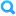## RubricFind Rubric
Keep in mind that 58 students have already been assessed using this rubric. Changing it will affect their evaluations.
Some Rubric (1)
Some Rubric (1)
Criteria Ratings Pts
1(a) Plot
threshold: pts
 3.0 to >0.0 pts Correct Plot blank 0.0 to >0 pts No plot blank_2
pts
3.0 pts
--
1(b) Plot
threshold: pts
 3.0 to >0.0 pts Correct Plot _3657 0.0 to >0 pts No plot _962
pts
3.0 pts
--
1(c) Plot
threshold: pts
 3.0 to >0.0 pts Correct Plot _2370 0.0 to >0 pts No plot _1422
pts
3.0 pts
--
1(d) Plot
threshold: pts
 3.0 to >0.0 pts Correct Plot _2443 0.0 to >0 pts No plot _1167
pts
3.0 pts
--
2(a)
threshold: pts
 3.0 to >2.0 pts Correctly calculated eigenvalues and eigenvectors, with work shown _8087 2.0 to >1.0 pts Correctly applied the methods for finding eigenvalues and eigenvectors, with small computational error _7330 1.0 to >0.0 pts Large logical or method error for calculating eigenvalues or eigenvectors, but significant effort _3189 0.0 to >0 pts No work shown _589
pts
3.0 pts
--
2(b)
threshold: pts
 3.0 to >2.0 pts Correctly calculated eigenvalues and eigenvectors, with work shown _3888 2.0 to >1.0 pts Correctly applied the methods for finding eigenvalues and eigenvectors, with small computational error _3512 1.0 to >0.0 pts Large logical or method error for calculating eigenvalues or eigenvectors, but significant effort _9667 0.0 to >0 pts No work shown _5368
pts
3.0 pts
--
2(c)
threshold: pts
 3.0 to >2.0 pts Correctly calculated eigenvalues and eigenvectors, with work shown _1781 2.0 to >1.0 pts Correctly applied the methods for finding eigenvalues and eigenvectors, with small computational error _6055 1.0 to >0.0 pts Large logical or method error for calculating eigenvalues or eigenvectors, but significant effort _5883 0.0 to >0 pts No work shown _2629
pts
3.0 pts
--
Total Points: 21.0 out of 21.0
 I'll write free-form comments when assessing students Remove points from rubric Don't post Outcomes results to Learning Mastery Gradebook Use this rubric for assignment grading Hide score total for assessment results
 I'll write free-form comments when assessing students Remove points from rubric Don't post Outcomes results to Learning Mastery Gradebook Use this rubric for assignment grading Hide score total for assessment results